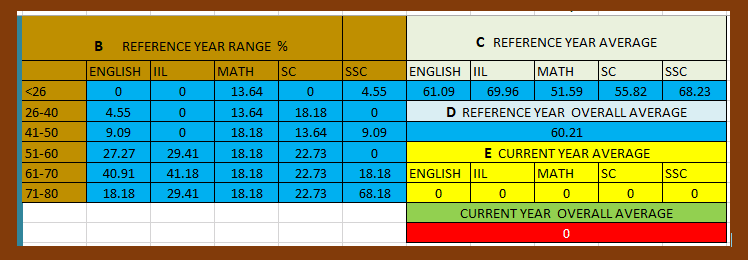CBSE has published the historic performance for CBSE 10th result 2021 format. In this article, we are going to provide you the CBSE 10th Result 2021 format based on Historic performance in an Excel file. So let’s start now!

Topics Covered

## CBSE 10th Result 2021 format based on Historic performance

This format is divided into 6 sections like Section A to F. Observe the screenshot given below:

## Marks Entry

1. Enter the reference year %, subject average, and overall average in Section B, C, and D.

2. Observe this screenshot.

## Formulas

In the next section of CBSE 10th Result 2021, I will explain the formulas used in this worksheet.

### How to write a formula to compute the current year average (section E)

Follow the below given steps to calculate the current year average marks:

1. Write the formula for English in O8 Cell: =SUM(B10:B34)/A35. In A35 cell I have calculated no. of students in the class using =COUNTA(A10:A34) formula.
2. Write formula for the respective subjects accordingly.

### Formula to calculate the current year overall average

=SUM(B10:F34)/(A35*5)

Select the cells starting from the subject 1 marks entry to last cell of subject 5 marks entry.

### Formula to calculate the range of allowed students

=ROUND(\$A\$35*J3/100,1)

A35 is the cell where the total no. of students is calculated. J3 is given % from the reference year in the given range. Write appropriate formula for all the subjects.

=COUNTIFS(B10:B34,”<26″)

This is the formal for calculating actual students ratio.

## Calculating 6th subject marks

For 6th Subject use this formula:

=((AVERAGE(LARGE(B10:F10,{1,2,3})))*50)/80

I hope this will helpful for you to make any changes to the worksheet. The sheet is already based on a formula. Just enter your marks do accordingly.